# Structure substitutive des pavages apériodiques de Jeandel-Rao¶

Sébastien Labbé, version 1 of arXiv:1808.07768, August 2018

Sébastien Labbé, version 2 of arXiv:1808.07768, April 2019

Sébastien Labbé, first online version in DCG, November 2019. We did changes to make the code work in Python 3 which is used in Sage starting from version 9.0.

Références:

## Prerequisites¶

Sage version 8.7 or above should work:

In :
version()

Out:
'SageMath version 9.0.beta6, Release Date: 2019-11-18'

The installation of the optional Sage package slabbe is a prerequisite. Remove the dash sign (#) and evaluate the following cell to install slabbe optional package.

The installation of graphviz and of the optional Sage package dot2tex is a prerequisite to construct the graphs at the end of the notebook.

Finally, I am using rise for the presentation and tikzmagic in Jupyter cells.

In :
#!sage -pip install slabbe>=0.4.4
#!sage -pip install dot2tex
#!sage -pip install rise
#!sage -pip install git+git://github.com/robjstan/tikzmagic.git


## Choosing a solver¶

The time it takes to evaluate all cells of this notebook depend on the chosen available solver.

Solver Type Time to run this notebook
Gurobi MILP solver about 4 minutes
Glucose SAT solver about 30 minutes (estimated)

The above timing were computed on a 2019 computer with 8 available cpus.

Dancing links is an algorithm proposed by D. Knuth to solve the Exact Cover Problem and is available in plain Sage.

Gurobi is a MILP solver. It is a proprietary software, but it is free for researchers and students. See this tutorial to make Gurobi available through Sage.

Glucose is a SAT solver developped at LaBRI (Bordeaux). It can be installed in sage>=8.7.beta0 easily:

sage -i glucose
In :
from sage.misc.package import is_package_installed
from sage.doctest.external import has_gurobi
if has_gurobi():
solver = 'gurobi'
elif is_package_installed('glucose'):
solver = 'glucose'
else:
solver = 'dancing_links' # more time efficient
print("We are using solver = '{}'".format(solver))

We are using solver = 'dancing_links'


# Setup¶

Colors setup:

In :
from collections import defaultdict
color = defaultdict(lambda : 'white')
color.update({0:'white', 1:'red', 2:'cyan', 3:'green', 4:'lightgray'})
color.update({str(k):v for k,v in color.items()})


If needed, here is the command to install tikzmagic:

sage -pip install git+git://github.com/robjstan/tikzmagic.git
In :
from slabbe import WangTileSet, Substitution2d
import tikzmagic


Latex macros:

$\newcommand{T}{{\mathcal{T}}}$ $\newcommand{U}{{\mathcal{U}}}$ $\newcommand{N}{{\mathbb{N}}}$ $\newcommand{Z}{{\mathbb{Z}}}$ $\newcommand{R}{{\mathbb{R}}}$

## Definition of Jeandel-Rao Tiles $\mathcal{T}_0$¶

In :
tiles = [(2,4,2,1), (2,2,2,0), (1,1,3,1), (1,2,3,2), (3,1,3,3), (0,1,3,1), (0,0,0,1), (3,1,0,2), (0,2,1,2), (1,2,1,4), (3,3,1,2)]
tiles = [[str(a) for a in t] for t in tiles]
T0 = WangTileSet(tiles)
T0.tikz(font=r'\small', size=1.2, ncolumns=11, color=color)

Out: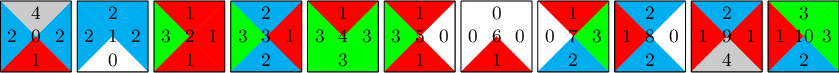Let $$\Omega_0=\Omega_{\T_0}=\{w:\Z\times\Z\to\T_0\mid w \text{ is a valid Wang tiling}\}$$ be the set of tilings made with the 11 Jeandel-Rao tiles.

In :
%time solution = T0.solver(30,15).solve(solver='glucose')

CPU times: user 248 ms, sys: 4 ms, total: 252 ms
Wall time: 478 ms

In :
solution.tikz(color=color)

Out: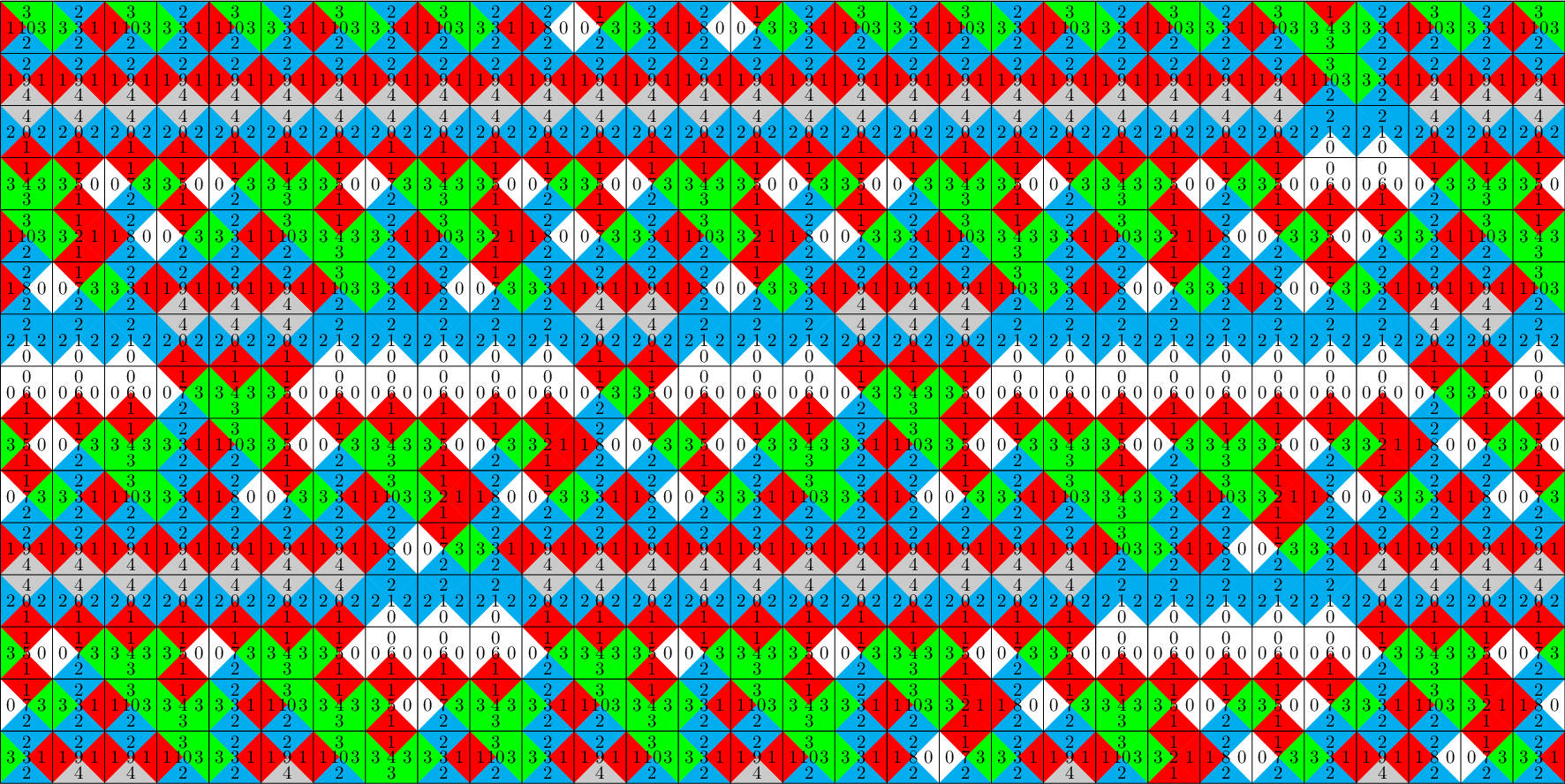The substitutive structure of Jeandel-Rao tilings $\Omega_0$ looks like this:

In :
%tikz -i subs-struct.tikz --no-wrap

Out:The current Jupyter notebook computes

• the Wang tile sets $\T_i$ for each $i\in\{1,\dots,12\}$
• the Wang shifts $\Omega_i=\Omega_{\T_i}=\{w:\Z\times\Z\to\T_i\mid w \text{ is a valid Wang tiling}\}$ for each $i\in\{1,\dots,12\}$
• the 2-dimensional morphisms $\omega_i:\Omega_{i+1}\to\Omega_i$ for each $i\in\{1,\dots,12\}\setminus\{4,5\}$
• the embedding $\pi:\Omega_5\to\Omega_4$
• the topological conjugacy $\eta:\Omega_6\to\Omega_5$

Algorithm.

INPUTS: $\T$ is a Wang tile set; $i\in\{1,2\}$ is a direction $e_i$; $r\in\N$ is some radius.

FindMarkers($\mathcal{T}$, $i$, $r$)

• $j\gets 3-i$
• $D_j \gets \left\{(u,v)\in\T^2\mid u\odot^jv\text{ admits a surrounding or radius } r\text{ in }\Omega_\T \right\}$
• $U \gets$UnionFindDataStructure$(\T)$
• For All $(u,v) \in D_j$
• Union$(u, v)$ (Merge $u$ and $v$ in the data structure $U$)
• EndFor
• $D_i \gets \left\{(u,v)\in\T^2\mid u\odot^iv \text{ admits a surrounding or radius } r \text{ in }\Omega_\T\right\}$
• Return ${S \in\textbf{Subsets}(U) \mid  \left(S\times S\right) \cap D_i=\varnothing\}$
• The output contains zero, one or more subsets of markers in the direction $i$.

## Computing $\mathcal{T}_1$¶

In :
T0.tikz(font=r'\small', ncolumns=11, size=1.2, color=color)

Out: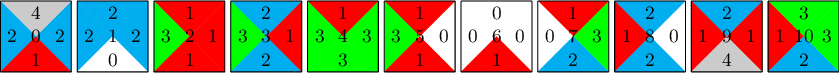In :
%time T0.find_markers(i=2, radius=1, solver=solver) # 229ms with dancing_links, 32s with Glucose, 1.4s with Gurobi

CPU times: user 260 ms, sys: 0 ns, total: 260 ms
Wall time: 258 ms

Out:
[[0, 1]]
In :
M0 = [0,1]
T1,omega0 = T0.find_substitution(M=M0, i=2, side='left', solver=solver)

In :
show(omega0)

In :
T1.tikz(font=r'\small', size=1.2, ncolumns=13)

Out: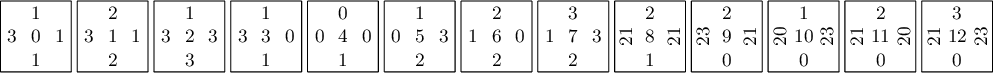## Computing $\mathcal{T}_2$¶

In :
T1.tikz(font=r'\small', ncolumns=20, size=1.2)

Out:In :
%time T1.find_markers(i=2, radius=1, solver=solver) #385ms with dancing_links, 46s with Glucose, 2s with Gurobi

CPU times: user 416 ms, sys: 0 ns, total: 416 ms
Wall time: 413 ms

Out:
[[8, 9, 10, 11, 12]]
In :
M1 = [8, 9, 10, 11, 12]
T2,omega1 = T1.find_substitution(M=M1, i=2, side='left', solver=solver)

In :
show(omega1)

In :
T2.tikz(font=r'\small', size=1.2, ncolumns=20)

Out: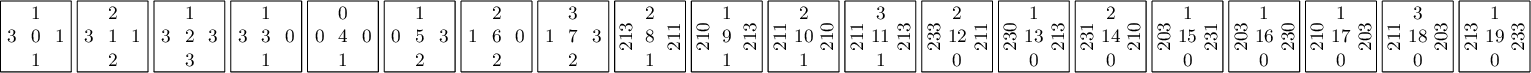## Computing $\mathcal{T}_3$¶

In :
T2.tikz(font=r'\small', size=1, ncolumns=20)

Out: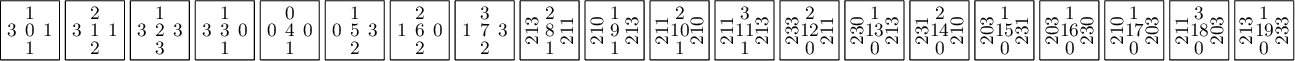In :
%time T2.find_markers(i=2, radius=2, solver=solver) # 4s with dancing_links, 2min 23s with Glucose, 15s with Gurobi

CPU times: user 4.04 s, sys: 0 ns, total: 4.04 s
Wall time: 4.04 s

Out:
[[8, 9, 10, 11, 12, 13, 14, 15, 16, 17, 18, 19]]
In :
M2 = [8, 9, 10, 11, 12, 13, 14, 15, 16, 17, 18, 19]
T3,omega2 = T2.find_substitution(M=M2, i=2, side='left', radius=2, solver=solver)

In :
show(omega2)

In :
T3.tikz(font=r'\small', size=1, ncolumns=24)

Out: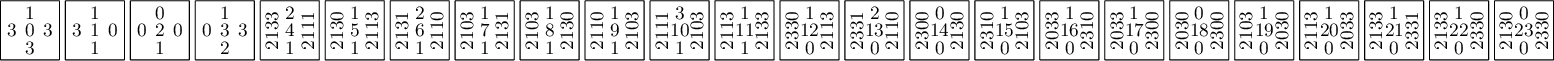## Computing $\mathcal{T}_4'$¶

In :
T3.tikz(font=r'\small', size=1, ncolumns=24)

Out: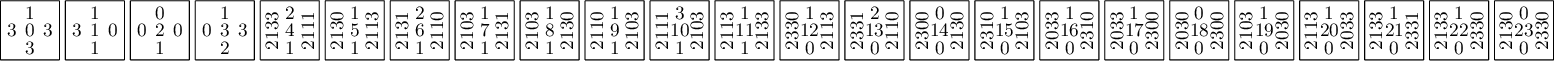In :
%time T3.find_markers(i=2, radius=3, solver=solver) # 13s with dancing_links, 4min 55s with Glucose, 59s with Gurobi

CPU times: user 14.8 s, sys: 0 ns, total: 14.8 s
Wall time: 14.8 s

Out:
[[0, 1, 2, 3]]
In :
M3 = [0, 1, 2, 3]
T4p,omega3p = T3.find_substitution(M=M3, i=2, side='right', radius=3, solver=solver)

In :
show(omega3p)

In :
T4p.tikz(font=r'\small', size=1.2, ncolumns=15)

Out: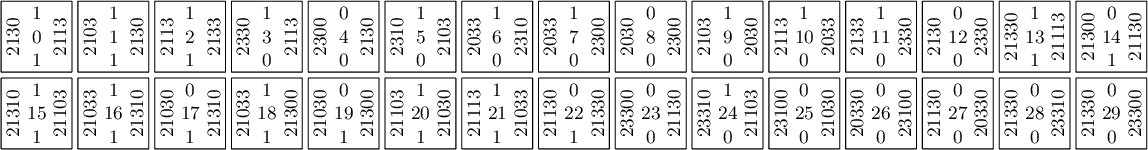## Check that we can remove 2 tiles (tiles #24 and #28) from $\mathcal{T}_4'$¶

In :
T4p.tikz(font=r'\small', size=1.2, ncolumns=15)

Out:We check that tiles #24 and #28 can be removed from T4p. Since tile #28 is always to the left of #24, it suffices to check that the tile #24 does not admit a large enough surrounding (here of width 71 and heigth 9).

Gurobi or Glucose can solve this in a reasonable amount of time (<6s):

In :
%%time
assert T4p == ('21103', '1', '23310', '0')
S = T4p.solver(width=71, height=9, preassigned_tiles={(35,4):24})
print(S.has_solution(solver='glucose'))          # 6s with Gurobi, 4s with Glucose

False
CPU times: user 2.48 s, sys: 60 ms, total: 2.54 s
Wall time: 4.43 s


## Computing $\mathcal{T}_4$¶

In :
forbidden = ('21103', '1', '23310', '0'), ('23310', '0', '21330', '0')
id_tiles = [(i,t) for (i,t) in enumerate(T4p) if t not in forbidden]
indices,tiles = zip(*id_tiles)
d = dict(enumerate(indices))
iota = Substitution2d.from_permutation(d)
T4 = WangTileSet(tiles)

In :
omega3 = omega3p * iota
show(omega3)

In :
T4.tikz(font=r'\small', size=1.2, ncolumns=15)

Out: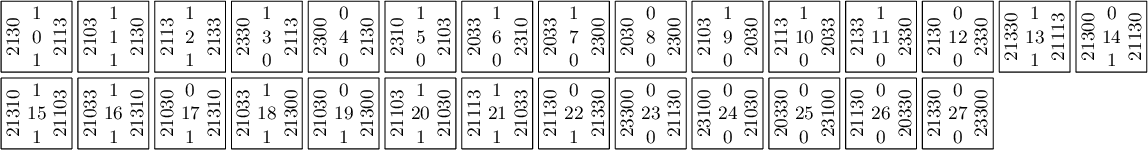## The morphism $\omega_0\omega_1\omega_2\omega_3:\Omega_{4}\to\Omega_{0}$¶

In :
omega0to4 = omega0*omega1*omega2*omega3
show(omega0to4)

In :
omega0to4.wang_tikz(T4, T0, font=r'\scriptsize', scale=.8, codomain_color=color, ncolumns=10, direction='down', extra_space=1.5)

Out: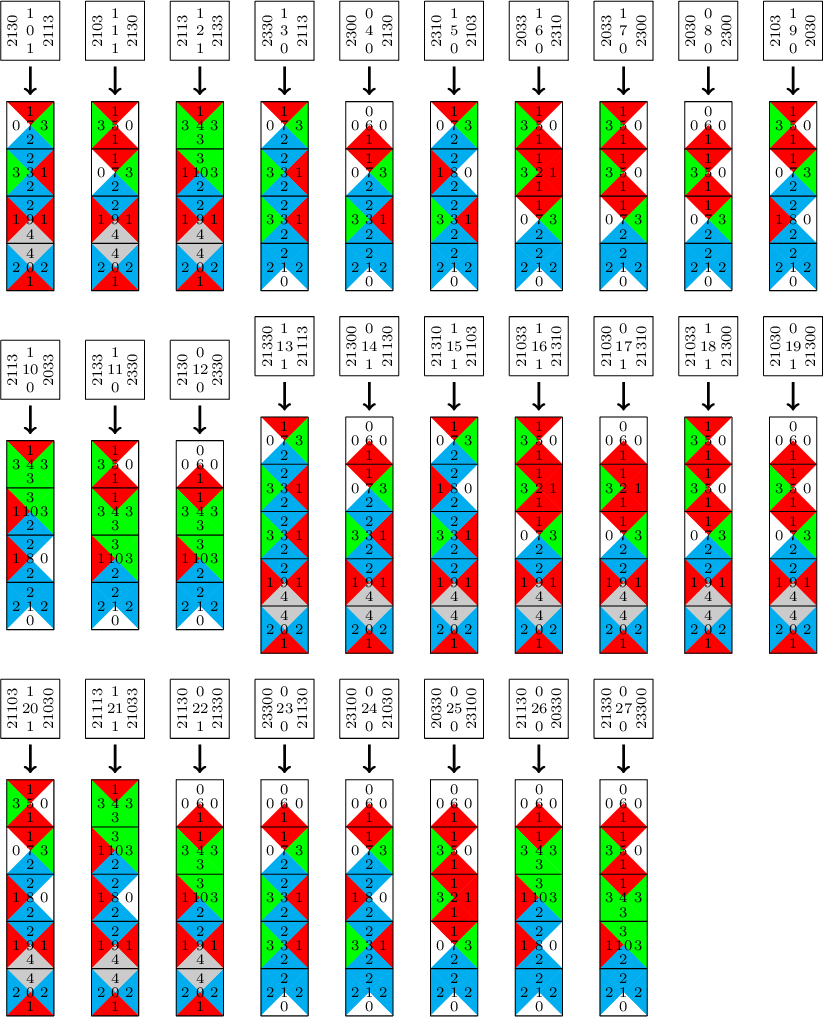## Trying to compute $\T_5$ ...¶

In :
T4.tikz(font=r'\small', size=1, ncolumns=15)

Out: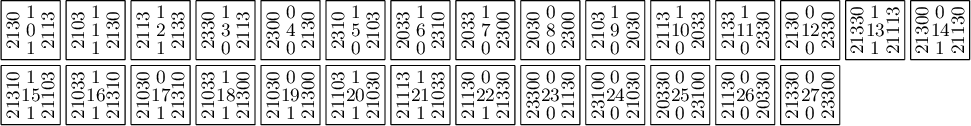In :
%time T4.find_markers(i=1, radius=1, solver=solver)

CPU times: user 4.12 s, sys: 0 ns, total: 4.12 s
Wall time: 4.12 s

Out:
[]
In :
%time T4.find_markers(i=2, radius=1, solver=solver)

CPU times: user 0 ns, sys: 0 ns, total: 0 ns
Wall time: 126 µs

Out:
[]

We have a problem: the Wang tile set $\T_4$ has markers neither in the direction $e_1$ nor $e_2$

So increasing the surrounding radius will not help here.

Even worse, we have two problems...

## Defining $\mathcal{T}_5$¶

The first problem is the presence of horizontal fracture lines which we can break by adding decorations on the horizontal edges of few tiles. We have to do it in a way that we forbid sliding along fracture lines and without destroying the overall structure of Wang tilings in $\Omega_4$.

We create the tile set $\mathcal{T}_5$ by adding decorations on $\mathcal{T}_4$.

For few tiles, the horizontal color 0 is replaced by color 6.

For few tiles, the horizontal color 1 is replaced by color 5.

In :
tiles5 = [('2113', '5', '2130', '1'),
('2130', '1', '2103', '5'),
('2133', '1', '2113', '1'),
('2113', '5', '2330', '0'),
('2130', '6', '2300', '0'),
('2103', '5', '2310', '0'),
('2310', '1', '2033', '6'),
('2300', '1', '2033', '6'),
('2300', '0', '2030', '6'),
('2030', '1', '2103', '0'),
('2033', '1', '2113', '0'),
('2330', '1', '2133', '6'),
('2330', '0', '2130', '6'),
('21113', '5', '21330', '1'),
('21130', '6', '21300', '1'),
('21103', '5', '21310', '1'),
('21310', '1', '21033', '5'),
('21310', '0', '21030', '5'),
('21300', '1', '21033', '5'),
('21300', '0', '21030', '5'),
('21030', '1', '21103', '1'),
('21033', '1', '21113', '1'),
('21330', '0', '21130', '1'),
('21330', '0', '21130', '5'),
('21130', '6', '23300', '0'),
('21030', '6', '23100', '0'),
('23100', '0', '20330', '6'),
('20330', '0', '21130', '0'),
('23300', '0', '21330', '6')]
T5 = WangTileSet(tiles5)
T5.tikz(font=r'\small', size=1.2, ncolumns=15)

Out: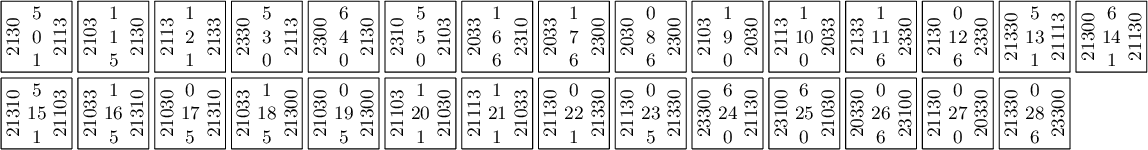## The morphism $\pi:\mathcal{T}_5\to\mathcal{T}_4$¶

The morphism $\pi$ erases the decorations on tiles of $\mathcal{T}_5$.

It projects the horizontal color 6 back to color 0, and the horizontal color 5 back to color 1.

On tiles, the map $\pi:\mathcal{T}_5\to\mathcal{T}_4$ is not injective as it maps two tiles (#22 and #23) onto the same (#22).

In :
def project_56(t, p56='10'):
L = []
d = dict(zip('56', p56))
for w in t:
for a in '56':
w = w.replace(a, d[a])
L.append(w)
return tuple(L)

In :
T4_tiles_list = T4.tiles()
d = {}
for i,t in enumerate(T5):
pt = project_56(t, p56='10')
j = T4_tiles_list.index(pt)
d[i] = j
omega4_pi = Substitution2d.from_permutation(d)
show(omega4_pi)


But surprise, on tilings, the map $\pi:\Omega_5\to\Omega_4$ defines a $2$-dimensional morphism which is an embedding, i.e., injective.

## Trying to compute $\T_6$ from $\T_5$ ...¶

In :
T5.tikz(font=r'\small', size=1, ncolumns=15)

Out: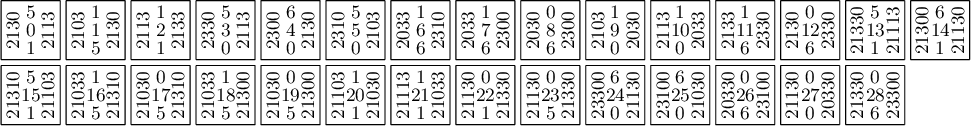In :
%time T5.find_markers(i=1, radius=1, solver=solver)

CPU times: user 4.74 s, sys: 4 ms, total: 4.74 s
Wall time: 4.74 s

Out:
[]
In :
%time T5.find_markers(i=2, radius=1, solver=solver)

CPU times: user 0 ns, sys: 0 ns, total: 0 ns
Wall time: 70.6 µs

Out:
[]

We still have a problem: the Wang tile set $\T_5$ has markers neither in the direction $e_1$ nor $e_2$

So increasing the surrounding radius will not help here.

## Tilings in $\Omega_5$¶

Let's look at tilings in $\Omega_5$.

In :
tiling = T5.solver(30,10).solve(solver='glucose')

In :
P = tiling.tile_positions([1, 6, 7, 8, 11, 12, 16, 17, 18, 19, 23, 26, 28])
s = '\n'.join([r'\fill[yellow] {} rectangle {};'.format((a,b), (a+1,b+1)) for (a,b) in P])
tiling.tikz(extra_before=s)

Out: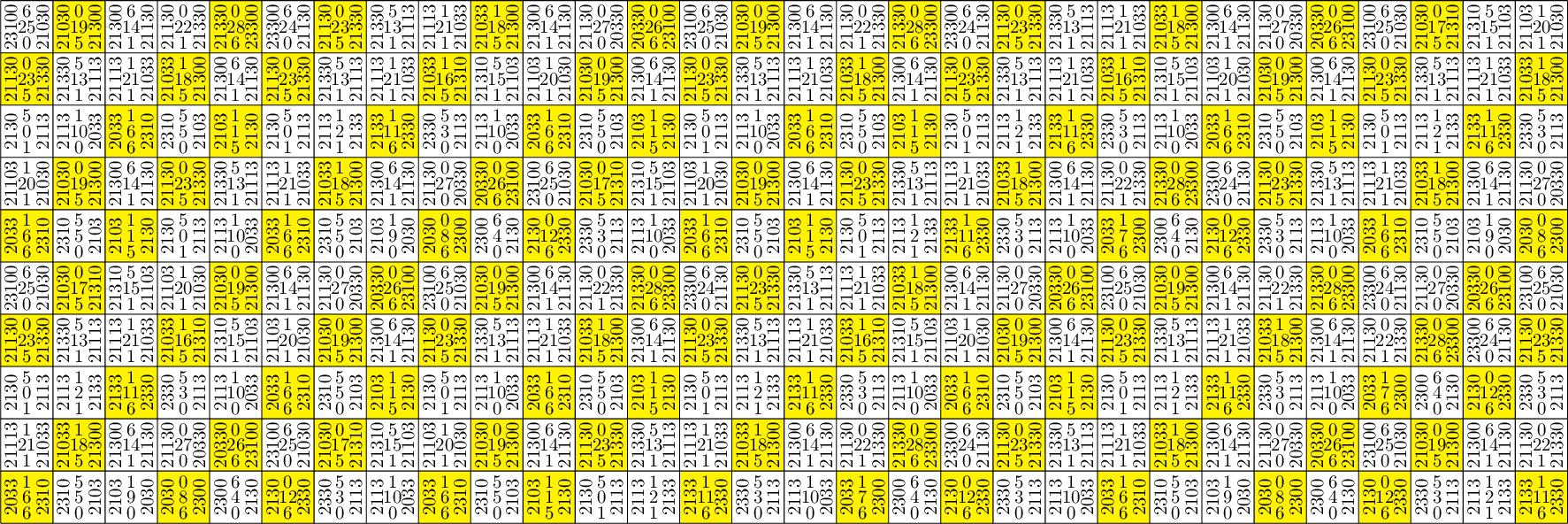## Computing $\mathcal{T}_6$ and the shear topological conjugacy $\eta:\Omega_6\to\Omega_5$¶

The second problem is that $\T_5$ does not have markers, it has diagonal markers, i.e., markers appears on lines of slope 1.

To fix this, instead of introducing the notion of diagonal markers, we choose to transform the Wang tile set in a way that it shears the tiling by the matrix $\left(\begin{smallmatrix} 1 & -1 \\ 0 & 1\end{smallmatrix}\right)$ so that markers become vertical.

In :
%tikz -i shear.tikz -p amssymb --no-wrap

Out:This transformation on tiles yields a homeomorphism $\eta:\Omega_6\to\Omega_5$ which commutes the normal shift on $\Omega_5$ into the sheared shift on $\Omega_6$.

In :
%time T6,omega5_sheer = T5.shear(radius=2, solver=solver) # 6s with dancing_links, 3min 12s with Glucose, 22s with Gurobi

CPU times: user 6.38 s, sys: 0 ns, total: 6.38 s
Wall time: 6.38 s

In :
show(omega5_sheer)

In :
T6.tikz(font=r'\small', size=1.2, ncolumns=15)

Out: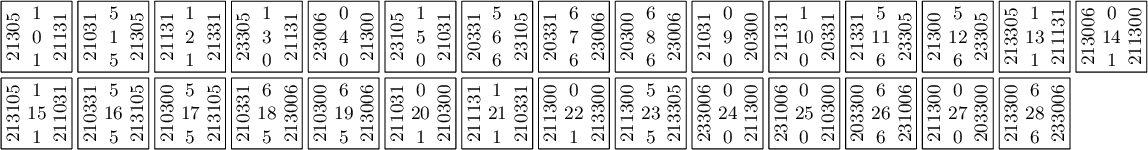## Computing $\mathcal{T}_7$¶

In :
T6.tikz(font=r'\small', size=1.2, ncolumns=15)

Out: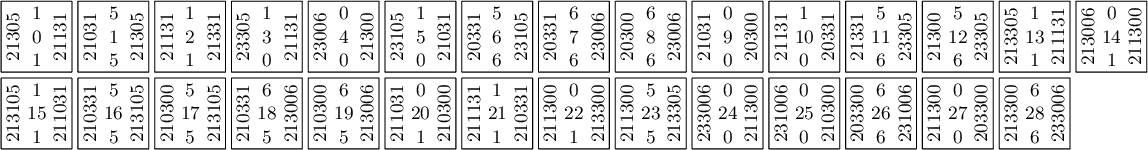In :
%time T6.find_markers(i=1, radius=1, solver=solver) # 5s with dancing_links, 4min 25s with Glucose, 17s with Gurobi

CPU times: user 4.79 s, sys: 4 ms, total: 4.8 s
Wall time: 4.8 s

Out:
[[0, 3, 4, 5, 13, 14, 15, 24, 25],
[1, 6, 7, 8, 11, 12, 16, 17, 18, 19, 23, 26, 28],
[2, 9, 10, 20, 21, 22, 27]]

Youpi! The tile set $\T_6$ has 3 subsets of markers in the direction $e_1$. Thus, we may chose one, desubstitute and compute $\T_7$.

In :
M6 = [1, 6, 7, 8, 11, 12, 16, 17, 18, 19, 23, 26, 28]
T7,omega6 = T6.find_substitution(M=M6, i=1, radius=1, side='left', solver=solver)

In :
show(omega4_pi*omega5_sheer*omega6)

In :
T7.tikz(font=r'\small', size=1.2)

Out: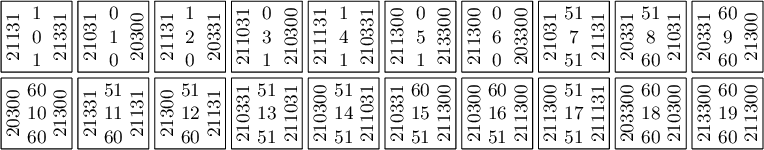## Computing $\mathcal{T}_8$¶

In :
T7.tikz(font=r'\small', size=1.2)

Out: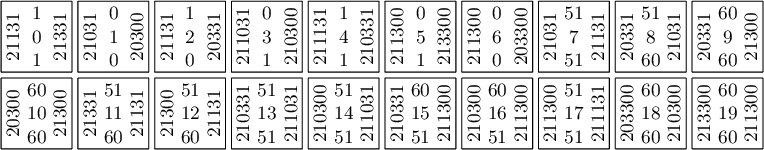In :
%time T7.find_markers(i=1, radius=1, solver=solver) # 2s with dancing_links, 2min with Glucose, 6s with Gurobi

CPU times: user 1.53 s, sys: 0 ns, total: 1.53 s
Wall time: 1.53 s

Out:
[[0, 1, 2, 3, 4, 5, 6]]
In :
M7 = [0, 1, 2, 3, 4, 5, 6]
T8,omega7 = T7.find_substitution(M=M7, i=1, radius=1, solver=solver)

In :
show(omega7)

In :
T8.tikz(font=r'\small', size=1.2)

Out: